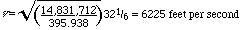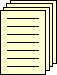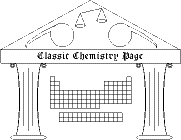## James Prescott Joule (1818-1889)

### Some Remarks on Heat and the Constitution of Elastic Fluids

Memoirs of the Manchester Literary and Philosophical Society November 1851 and Philosophical Magazine 14, 211 (1857) reprinted in William Francis Magie, ed., A Source Book in Physics (New York: McGraw-Hill, 1935)

I have myself endeavoured to prove that a rotary motion, such as that described by Sir H. Davy, can account for the law of Boyle and Mariotte, and other phenomena presented by elastic fluids; nevertheless, since the hypothesis of Herapath--in which it is assumed that the particles of a gas are constantly flying about in every direction with great velocity, the pressure of the gas being owing to the impact of the particles against any surface presented to them--is somewhat simpler, I shall employ it in the following remarks on the constitution of elastic fluids, premising, however, that the hypothesis of a rotary motion accords equally well with the phenomena.

Let us suppose an envelope of the size and shape of a cubic foot to be filled with hydrogen gas, which, at 60° [Fahrenheit--CJG] temperature and 30 inches barometrical pressure, will weigh 36.927 grs. [grains, not grams--CJG] Further, let us suppose the above quantity to be divided into three equal and indefinitely small elastic particles, each weighing 12.309 grs.; and, further, that each of these particles vibrates between opposite sides of the cube, and maintains a uniform velocity except at the instant of impact; it is required to find the velocity at which each particle must move so as to produce the atmospherical pressure of 14,831,712 grs. on each of the sides of the cube. In the first place, it is known that if a body moving with the velocity of 32 1/6 feet per second be opposed, during one second, by a pressure equal to its weight its motion will be stopped, and that, if the pressure be continued one second longer, the particle will acquire the velocity of 32 1/6 feet per second in a contrary direction. At this velocity there will be 32 1/6 collisions of a particle of 12.309 grs. against each side of the cubical vessel in every two seconds of time; and the pressure occasioned thereby will be 12.309 x 32 1/6 = 395.938 grs. Therefore, since it is manifest that the pressure will be proportional to the square of the velocity of the particles, we shall have for the velocity of the particles requisite to produce the pressure of 14,831,712 grs. on each side of the cubical vessel,The above velocity will be found equal to produce the atmospheric pressure, whether the particles strike each other before they arrive at the sides of the cubical vessel, whether they strike the sides obliquely, and, thirdly, into whatever number of particles the 36.927 grs. of hydrogen are divided.

If only one half the weight of hydrogen, or 18.4635 grs., be enclosed in the cubical vessel, and the velocity of the particles be, as before, 6225 feet per second, the pressure will manifestly be only one half of what it was previously; which shows that the law of Boyle and Mariotte flows naturally from the hypothesis.

The velocity above named is that of hydrogen at the temperature of 60°; but we know that the pressure of an elastic fluid at 60° is to that at 32° as 519 is to 491. Therefore the velocity of the particles at 60° will be to that at 32° as sqrt(519):sqrt(491); which shows that the velocity at the freezing temperature of water is 6055 feet per second.

In the above calculations it is supposed that the particles of hydrogen have no sensible magnitude, otherwise the velocity corresponding to the same pressure would be lessened.

Since the pressure of a gas increases with its temperature in arithmetical progression, and since the pressure is proportional to the squares of the velocity of the particles, in other words to their vis viva, it follows that the absolute temperature, pressure, and vis viva are proportional to one another, and that the zero of temperature is 491° below the freezing-point of water. Further, the absolute heat of the gas, or, in other words, its capacity, will be represented by the whole amount of vis viva at a given temperature. The specific heat may therefore be determined in the following simple manner:--

The velocity of the particles of hydrogen, at the temperature of 60°, has been stated to be 6225 feet per second, a velocity equivalent to a fall from the perpendicular height of 602,342 feet. The velocity at 61° will be 6225xsqrt(520/519) = 6230.93 feet per second, which is equivalent to a fall of 603,502 feet. The difference between the above falls is 1160 feet, which is therefore the space through which 1 lb. of pressure must operate upon each lb. of hydrogen, in order to elevate its temperature one degree. But our mechanical equivalent of heat shows that 770 feet is the altitude representing the force required to raise the temperature of water one degree; consequently the specific heat of hydrogen will be 1160/778 = 1.506, calling that of water unity.

The specific heats of other gases will be easily deduced from that of hydrogen; for the whole vis viva and capacity of equal bulks of the various gases will be equal to one another; and the velocity of the particles will be inversely as the square root of the specific gravity. Hence the specific heat will be inversely proportional to the specific gravity, a law which has been arrived at experimentally by De la Rive and Marcet.Back to the list of selected historical papersBack to the top of the Classic Chemistry site